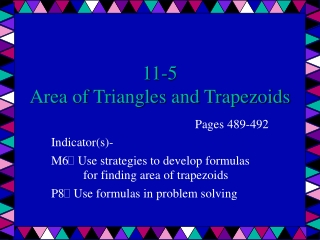DownloadDownload Presentation11-5 Area of Triangles and Trapezoids

# 11-5 Area of Triangles and Trapezoids

Télécharger la présentation## 11-5 Area of Triangles and Trapezoids

- - - - - - - - - - - - - - - - - - - - - - - - - - - E N D - - - - - - - - - - - - - - - - - - - - - - - - - - -
##### Presentation Transcript

1. 11-5Area of Triangles and Trapezoids Pages 489-492 Indicator(s)- M6 Use strategies to develop formulas for finding area of trapezoids P8 Use formulas in problem solving

2. Area of Triangles A=½bh • A triangle is a three-sided polygon. • The area of a triangle can be defined by half of the product of the length of its base and its height. The height is always perpendicular to the base, exactly like the base and height of a parallelogram. • A=½bh

3. Example • Find the area of the triangle below. Round to the nearest tenth if necessary. • A=½bh • A=½(9)(3.2) • A=½(28.8) • A= 14.4 cm2 • The area of the given triangle is 14.4cm2 3.2 cm 9 cm.

4. Area of Trapezoids A=½h(b1+b2) • A trapezoid has two (2) bases, b1&b2. The height of the trapezoid is the perpendicular distance between the two bases. • A=½h(b1+b2) b1 h b2

5. Example • Find the area of the trapezoid below. Round to the nearest tenth if necessary. • A=½h(b1+b2) • A=½(3)(4 + 7.6) • A=½(3)(11.6) • A= 17.4 m2 • The area of the given trapezoid is 17.4cm2 4 m 3 m 7.6 m

6. Example • The shape of the state of Montana resembles a trapezoid. Estimate its area in square miles. • A=½h(b1+b2) • A=½(285)(542 + 479) • A=½(285)(1021) • A≈ 145,493 mi2 • The area of the Montana is about 145,493 mi2. 542 mi 285 mi 479 mi

7. What if… you are dealing with two different measurements? Find the area of the given triangle. Round to the nearest tenth if necessary. • A=½bh • A=½(13 in)(1 ft) • CONVERT & SOLVE! • A=½(13 in)(12 in) • A= 78 in2 1 ft 13 in

8. Challenge… • Find the area of the trapezoid shown. Round to the nearest tenth if necessary. • A=½h(b1+b2) • HINT: To find the height, you will have to use the Pythagorean Theorem. 9cm 10 cm 6 cm